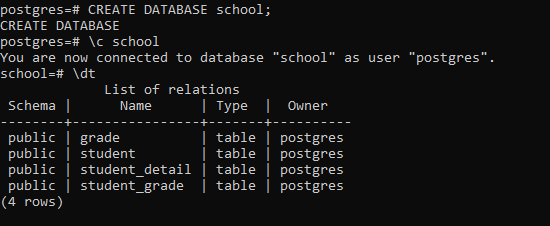# PostgreSQL – Create table using Python

• Last Updated : 30 Aug, 2020

This article explores the process of creating table in The PostgreSQL database using Python.

### Prerequisites:

• psycopg2 module
• sample database

### Creating a Table:

To create a table in the database use the following steps:

• First create a CREATE TABLE statement
• Second establish a connection to the database using the connect() function
• Third construct a cursor object by using the cursor() method.
• Now execute the above created CREATE TABLE statement using the execute function.

Example:

In this example we have already created a Database called school. We will be adding tables to it. To do so we created a file called create_table.py and defined a create_table() function as shown below:

## Python3

 `import` `psycopg2``from` `config ``import` `config`` ` ` ` `def` `create_tables():``    ``""" create tables in the PostgreSQL database"""``    ``commands ``=` `(``        ``"""``        ``CREATE TABLE student (``            ``student_id SERIAL PRIMARY KEY,``            ``student_name VARCHAR(255) NOT NULL``        ``)``        ``"""``,``        ``""" CREATE TABLE grade (``                ``grade_id SERIAL PRIMARY KEY,``                ``grade_name VARCHAR(255) NOT NULL``                ``)``        ``"""``,``        ``"""``        ``CREATE TABLE student_grade (``                ``grade_id INTEGER PRIMARY KEY,``                ``file_extension VARCHAR(5) NOT NULL,``                ``drawing_data BYTEA NOT NULL,``                ``FOREIGN KEY (grade_id)``                ``REFERENCES grade (grade_id)``                ``ON UPDATE CASCADE ON DELETE CASCADE``        ``)``        ``"""``,``        ``"""``        ``CREATE TABLE student_detail (``                ``student_id INTEGER NOT NULL,``                ``grade_id INTEGER NOT NULL,``                ``PRIMARY KEY (student_id , grade_id),``                ``FOREIGN KEY (student_id)``                    ``REFERENCES student (student_id)``                    ``ON UPDATE CASCADE ON DELETE CASCADE,``                ``FOREIGN KEY (grade_id)``                    ``REFERENCES grade (grade_id)``                    ``ON UPDATE CASCADE ON DELETE CASCADE``        ``)``        ``"""``)``    ``conn ``=` `None``    ``try``:``        ``# read the connection parameters``        ``params ``=` `config()``        ``# connect to the PostgreSQL server``        ``conn ``=` `psycopg2.connect(``*``*``params)``        ``cur ``=` `conn.cursor()``        ``# create table one by one``        ``for` `command ``in` `commands:``            ``cur.execute(command)``        ``# close communication with the PostgreSQL database server``        ``cur.close()``        ``# commit the changes``        ``conn.commit()``    ``except` `(Exception, psycopg2.DatabaseError) as error:``        ``print``(error)``    ``finally``:``        ``if` `conn ``is` `not` `None``:``            ``conn.close()`` ` ` ` `if` `__name__ ``=``=` `'__main__'``:``    ``create_tables()`

This will successfully create the tables :

• student
• student_detail

To verify so use the below command through the client tool of the same database(ie, school):

```\dt
```

Output:My Personal Notes arrow_drop_up